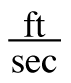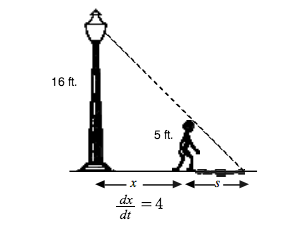### Home > CALC > Chapter 7 > Lesson 7.2.2 > Problem7-71

7-71.

Remember Eric and the 16-foot tall lamppost? If Eric (who is 5 feet tall) walks away from the pole at a rate of 4, at what rate is the tip of his shadow moving away from the lamppost? Draw a diagram before you start this problem. Homework Help ✎Find similar triangles and write a geometric equation relating their proportional sides.

Use implicit differentiation to convert the geometric equation into a rate equation.
In other words, find the derivative of everything in terms of time, t.